Euler's-Cauchy Method using Mathematica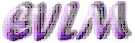Аnotation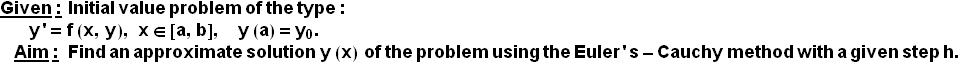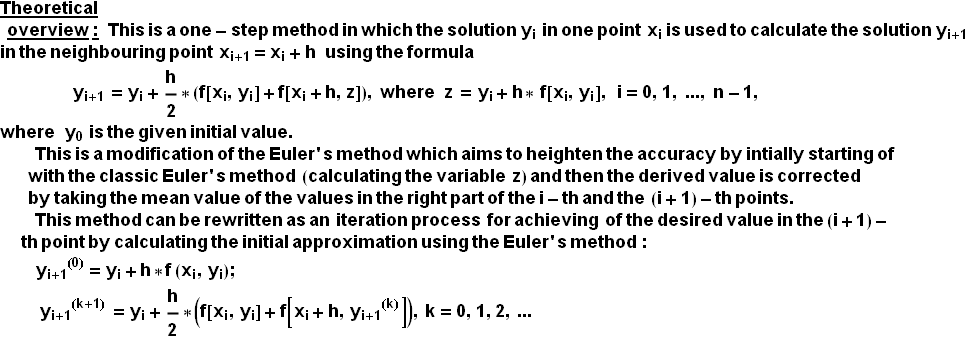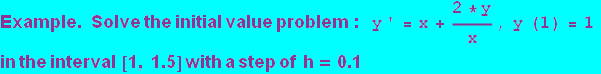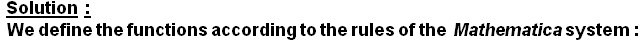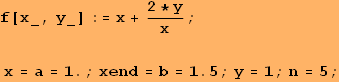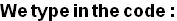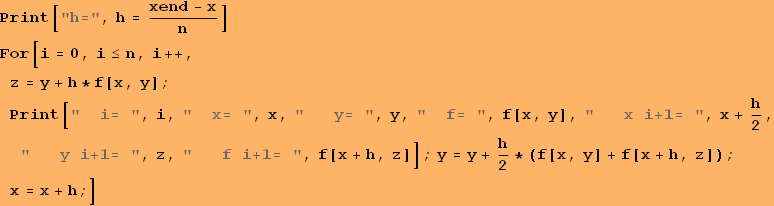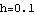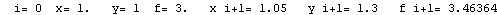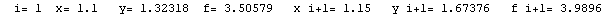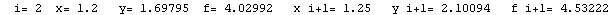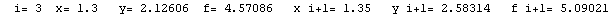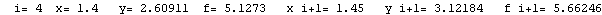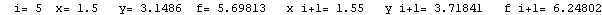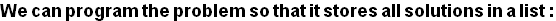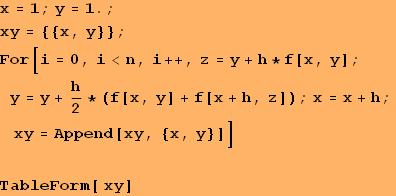1 1 1.1 1.32318 1.2 1.69795 1.3 2.12606 1.4 2.60911 1.5 3.1486

Conclusion:  The solution derived using the modified Euler's-Cauchy method is in the form of a table of the variable function y(x), as shown in the second column of the table above. Since the global apriori error of the method is О(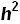) and here h=0.1, then these solutions must be rounded to only two signs after the decimal point.

Graphic of the solution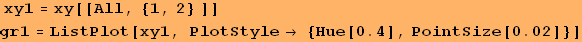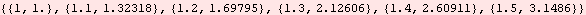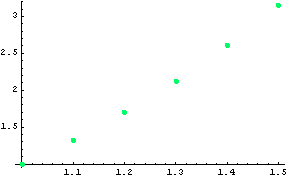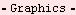Created by Wolfram Mathematica 6.0  (22 September 2008)